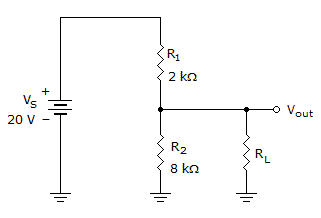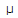# Electronics - Series-Parallel Circuits - Discussion

### Discussion :: Series-Parallel Circuits - General Questions (Q.No.10)

10.If the load in the given circuit is 80 k, what is the bleeder current?

 [A]. 196A [B]. 1.96 mA [C]. 2 mA [D]. 2.16 mA

Explanation:

No answer description available for this question.

 Sanj said: (Dec 30, 2010) Find the total resistance which will be = 9.2kohm Total current is =2.15mV Bleeder current is current through resistor R2 which is= 1.96mA Use the formula current through r2= rL/(r2+rL) (80/88)*2.15 = 1.95454545

 V.Nagendran said: (Sep 10, 2014) Can you please the Bleeder current?

 Faisal Amin said: (Jul 10, 2015) Here bleeder current is the current on R2 in the absence of load resistor RL. It means bleeder current is the unloaded current on the specific resistor where load is to be connected.

 Ameer said: (Dec 24, 2016) Nice @Sanj.

 Paresh said: (Sep 16, 2017) Why we are use 88? How to come please anyone give me explanation?

 Manoj said: (Oct 5, 2018) Here, RL + R2 = 88.